# Fuzzy C Means Clustering

Betul Mescioglu

## Fuzzy C Means Clustering

FCM is an unsupervised clustering technique that allows us to partition the data points based on their degree of membership to the clusters. In traditional (hard partitioning) clustering techniques, a data point is assigned to a certain cluster. It either belongs to that cluster or not. In FCM, each data point's probability of belonging to every one of the clusters is calculated. This method gives better results than k-means especially when it comes to overlapped data sets.For pre-clustering assessment, we will use the following vat() and ivat() functions which can be found in pyclustertend library.

``````import numpy as np
from sklearn.metrics import pairwise_distances
import matplotlib.pyplot as plt

def vat(data, return_odm=False, figure_size=(10, 10)):
"""VAT means Visual assesement of tendency. basically, it allow to asses cluster tendency
through a map based on the dissimiliraty matrix.

Parameters
----------

data : matrix
numpy array

return_odm : return the Ordered Dissimalirity Matrix
boolean (default to False)

figure_size : size of the VAT.
tuple (default to (10,10))

Return
-------

ODM : matrix
the ordered dissimalarity matrix plotted.

"""

ordered_dissimilarity_matrix = compute_ordered_dissimilarity_matrix(data)

_, ax = plt.subplots(figsize=figure_size)
ax.imshow(ordered_dissimilarity_matrix, cmap='gray', vmin=0, vmax=np.max(ordered_dissimilarity_matrix))

if return_odm is True:
return ordered_dissimilarity_matrix

def compute_ordered_dissimilarity_matrix(X):
"""The ordered dissimilarity matrix is used by visual assesement of tendency. It is a just a a reordering
of the dissimilarity matrix.

Parameters
----------

X : matrix
numpy array

Return
-------

ODM : matrix
the ordered dissimalarity matrix .

"""

# Step 1 :

observation_path = []

matrix_of_pairwise_distance = pairwise_distances(X)
list_of_int = np.zeros(matrix_of_pairwise_distance.shape, dtype="int")

index_of_maximum_value = np.argmax(matrix_of_pairwise_distance)

column_index_of_maximum_value = index_of_maximum_value // matrix_of_pairwise_distance.shape

list_of_int = column_index_of_maximum_value
observation_path.append(column_index_of_maximum_value)

K = np.linspace(0, matrix_of_pairwise_distance.shape - 1, matrix_of_pairwise_distance.shape, dtype="int")
J = np.delete(K, column_index_of_maximum_value)

# Step 2 :

for r in range(1, matrix_of_pairwise_distance.shape):

p, q = (-1, -1)

mini = np.max(matrix_of_pairwise_distance)

for candidate_p in observation_path:
for candidate_j in J:
if matrix_of_pairwise_distance[candidate_p, candidate_j] < mini:
p = candidate_p
q = candidate_j
mini = matrix_of_pairwise_distance[p, q]

list_of_int[r] = q
observation_path.append(q)

ind_q = np.where(np.array(J) == q)
J = np.delete(J, ind_q)

# Step 3

ordered_matrix = np.zeros(matrix_of_pairwise_distance.shape)

for column_index_of_maximum_value in range(ordered_matrix.shape):
for j in range(ordered_matrix.shape):
ordered_matrix[column_index_of_maximum_value, j] = matrix_of_pairwise_distance[
list_of_int[column_index_of_maximum_value], list_of_int[j]]

# Step 4 :

return ordered_matrix

def ivat(data, return_odm=False, figure_size=(10, 10)):
"""iVat return a visualisation based on the Vat but more reliable and easier to
interpret.

Parameters
----------

data : matrix
numpy array

return_odm : return the Ordered Dissimalirity Matrix
boolean (default to False)

figure_size : size of the VAT.
tuple (default to (10,10))

Return
-------

D_prim : matrix
the ivat ordered dissimalarity matrix.

"""

ordered_matrix = compute_ivat_ordered_dissimilarity_matrix(data)

_, ax = plt.subplots(figsize=figure_size)
ax.imshow(ordered_matrix, cmap='gray', vmin=0, vmax=np.max(ordered_matrix))

if return_odm is True:
return ordered_matrix

def compute_ivat_ordered_dissimilarity_matrix(X):
"""The ordered dissimilarity matrix is used by ivat. It is a just a a reordering
of the dissimilarity matrix.

Parameters
----------

X : matrix
numpy array

Return
-------

D_prim : matrix
the ordered dissimalarity matrix .

"""

ordered_matrix = compute_ordered_dissimilarity_matrix(X)
re_ordered_matrix = np.zeros((ordered_matrix.shape, ordered_matrix.shape))

for r in range(1, ordered_matrix.shape):
# Step 1 : find j for which D[r,j] is minimum and j in [1:r-1]

j = np.argmin(ordered_matrix[r, 0:r])

# Step 2 :

re_ordered_matrix[r, j] = ordered_matrix[r, j]

# Step 3 : pour c : 1,r-1 avec c !=j
c_tab = np.array(range(0, r))
c_tab = c_tab[c_tab != j]

for c in c_tab:
re_ordered_matrix[r, c] = max(ordered_matrix[r, j], re_ordered_matrix[j, c])
re_ordered_matrix[c, r] = re_ordered_matrix[r, c]

return re_ordered_matrix
``````
``````#import necessary libraries:
import glob
import pandas as pd
import numpy as np
import skimage.io
import matplotlib.pyplot as plt
import warnings
warnings.filterwarnings("ignore")
from fcmeans import FCM
# absolute path to search all PMG files inside a specific folder
path = r'*.PGM'
files = glob.glob(path)``````
```C:\Users\h\anaconda3\lib\site-packages\pandas\core\computation\expressions.py:20: UserWarning: Pandas requires version '2.7.3' or newer of 'numexpr' (version '2.7.1' currently installed).
from pandas.core.computation.check import NUMEXPR_INSTALLED
```
``files``
```['4cov.PGM',
'No_Clust.PGM',
'No_Clust_nois_pt_01.PGM',
'Three_Close_Clust.PGM',
'Three_Close_Clust_nois_pt005.PGM',
'Two_Clus_Diff_Density.PGM',
'Two_Clus_Diff_Size.PGM',
'Two_Ellip_Diff_Density.PGM',
'Two_Ellip_Diff_Size.PGM',
'Two_Separat_Clust.PGM',
'Two_Separat_Clust_nois_pt005.PGM']```
``````#Show one of the images
skimage.io.imshow("Two_Clus_Diff_Density.PGM", cmap='gray')``````
`<matplotlib.image.AxesImage at 0x162851380d0>`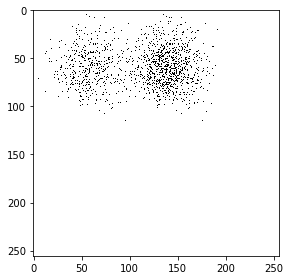The image has black dots on a white background. Black dots are going to be investigated by the algorithm, so, we need to create an array where black dots have the value of 1 and white pixels have the value of 0 as they are irrelevant. Then, we will apply ivat() function to the obtained array.

The fuzzy algorithm requires data points' locations. The following function detects the data points' coordinates in the image (dataset) and returns them as an array.

``````def extract_points(im):

X = []

for i in range(len(im)):
for j in range(len(im[i])):
if im[i][j]==1:
X.append([j,i])
return np.array(X)
``````

The following function applies fuzzy c means algorithm to the data points and plots the original image (dataset) and the resulting image with color coded clusters (with marked cluster centroids) for given values of number of clusters (n) and fuzzifier (q) number. It also saves these images.

``````def apply_fcm(X, n, q, name):
my_model = FCM(n_clusters=n, m=q)
my_model.fit(X)

centers = my_model.centers
labels = my_model.predict(X)
f, axes = plt.subplots(1, 2, figsize=(11,5))
axes.scatter(X[:,0], X[:,1], alpha=.9)
axes.set_title("Original Image")
axes.scatter(X[:,0], X[:,1], c=labels, alpha=.9)
axes.scatter(centers[:,0], centers[:,1], marker="+", s=500, c='r')
axes.set_title(f"Number of clusters: {n} Fuzzifier: {q}")
title = "Number_of_clusters _{}_Fuzzifier_{}_for_{}.png".format(n, q, name)
f.savefig(title)``````

### Image 1:

``````im1 = skimage.io.imread(files)
im1 = np.where(im1==255, 0, 1)
skimage.io.imshow(im1, cmap='gray')``````
`<matplotlib.image.AxesImage at 0x162851e8ac0>`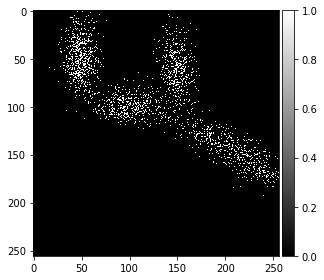``ivat(im1)``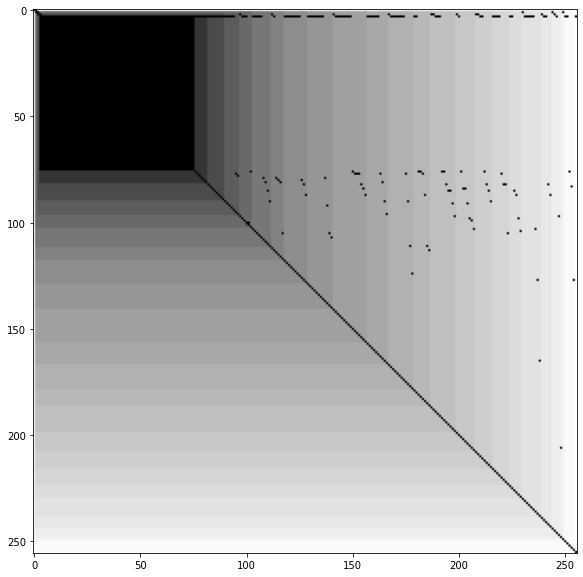In the image, all data points look like one big cluster. So, the map shows one dominant black square. However, we can also see the 4 groups in the map represented by the squares a little bigger than the black square. The colors start fading after the first 4 squares.

``````#Extract the coordinates of the data points
X = extract_points(im1)
#Apply fcm with 3, 4, 6 clusters and q values of 2, 5, 10.
for n in [3, 4, 6]:
for q in [2, 5, 10]:
apply_fcm(X, n, q, "Image1")``````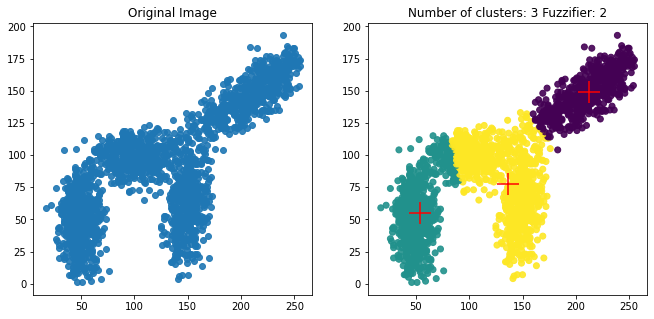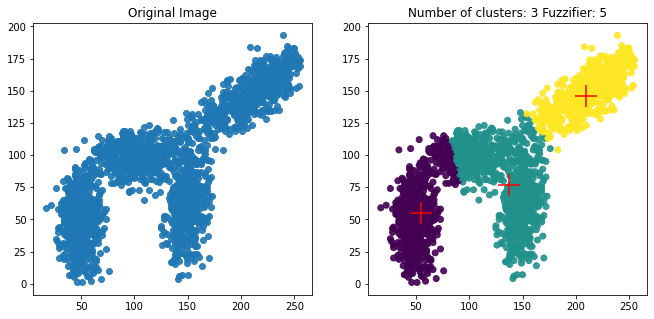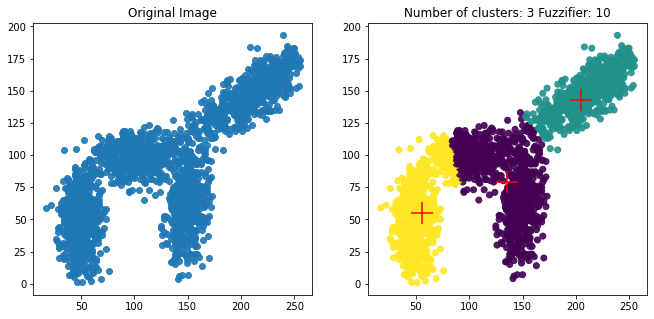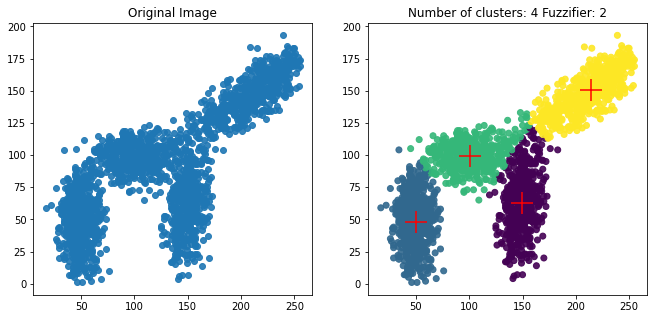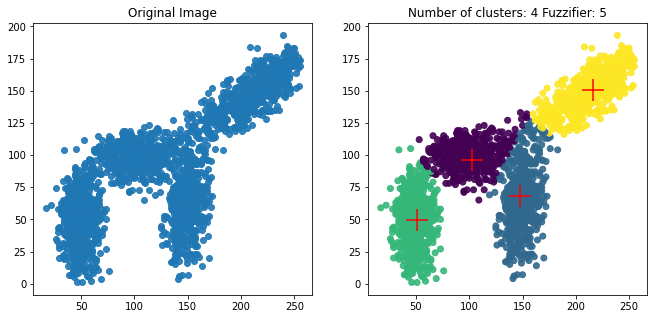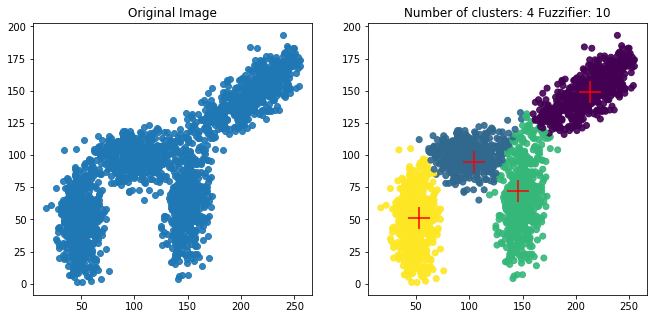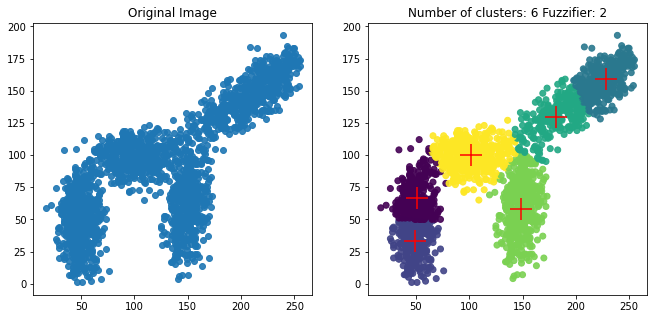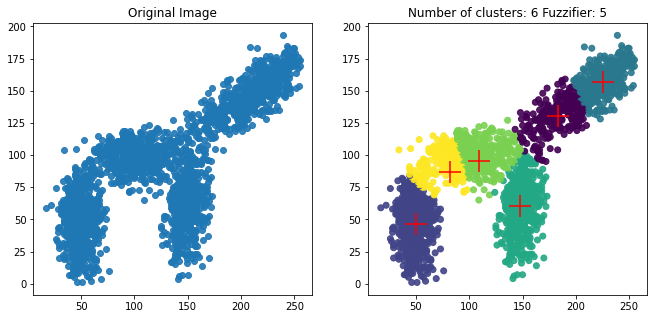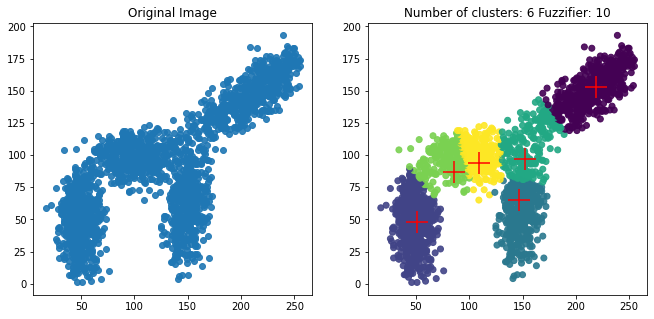From the ivat map, we observed that the data would render four clusters. From the FCM images, we see that when cluster number is four and fuzzifier is selected as two, the centroids are placed right in the middle of clusters and farther apart from each other. I would choose this one as outliers are identified correctly as well.

### Image 2:

``````im2 = skimage.io.imread(files)
im2 = np.where(im2==255, 0, 1)
skimage.io.imshow(im2, cmap='gray')``````
`<matplotlib.image.AxesImage at 0x162853731f0>`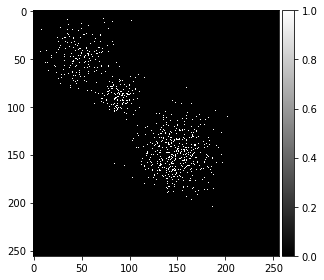``ivat(im2)``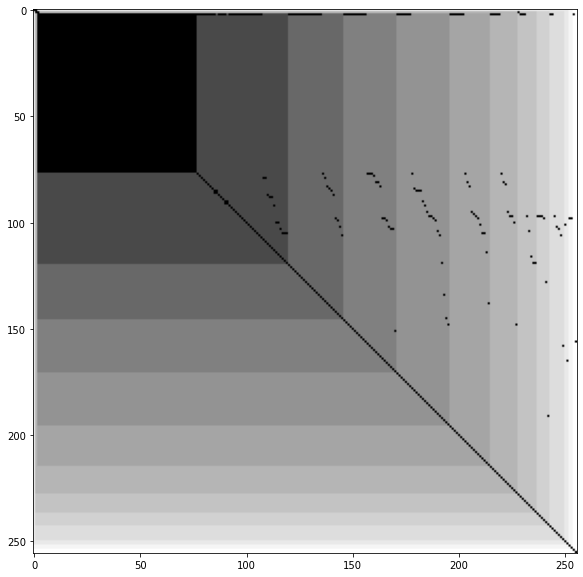Here again, we see three prominent squares nested in each other corresponding to the three clusters seen in the dataset.

``````X = extract_points(im2)
for n in [3, 4, 6]:
for q in [2, 5, 10]:
apply_fcm(X, n, q, "Image2")``````